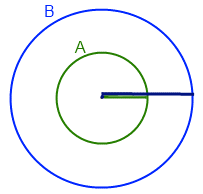+91-22-62437100 / 01 | help@reachivy.com

# SAT Math Quiz 1

Posted on Oct 16, 2017

To begin click Next

Question 1: Franklin bought several kites at the store, and each kite cost 16 dollars. Richard purchased several different kites, and spent 20 dollars each. If the ratio of the number of kites Franklin purchased to the number of kites Richard purchased is 3 to 2, what is the average cost of a kite purchased by Richard and Franklin?
Question 2:

Cecilia, Robbie, and Briony all bought stamps. The number of stamps Cecilia purchased was equal to a single digit. The number of stamps only one of them purchased was divisible by 3. The number of stamps one of them bought was an even number. Which of the following could represent the numbers of stamps each purchased?

Question 3:Circle A is inside Circle B, and the two circles share the same center O. If the circumference of B is four times the circumference of A, and the radius of Circle A is three, what is the difference between Circle B’s diameter and Circle A’s diameter?
Question 4:

A delivery cart went from Candleford to Lark Rise and back at an average speed of 2/3 miles per hour. If the distance from Candleford to Lark Rise is 1 mile, and the trip back took half as much time as the trip there, what was the average speed of the delivery cart on the way to Lark Rise?

Question 5:

In the figure, the radius of circle B is three-fourths the radius of circle A. The distance from center A to the circumference of circle B is 3, and the distance from center B to the circumference of circle A is 2. What is the area of the smaller circle?

Question 6:

The midpoints of the sides of a square are connected to form a new inscribed square. How many times greater than the area of the inscribed square is the area of the original square?

Question 7:

The diameter of a circle is increased by 80%. By what percent, approximately, is the area increased?

Question 8:

Shauna is throwing a bachelorette party for her best friend with x guests total. All of the guests plan to split the cost of renting a limo for y dollars. The day before, z guests cancel. Which of the following represents the percent increase in the amount each guest must pay towards the limo rental?

Question 9:

For all negative integers m other than -1, let ⏀m⏀ be defined as the product of all the negative odd integers greater than m. What is the value of ⏀-85⏀/⏀-84⏀?

Question 10:

On a number line with points LMNOP, the ratio of LM to MN is 1, and the ratio of NO to OP is 3/4. If the length of LP is 28 and the length of MO is 13, what is the ratio of LO to MP?

You have answered all the questions in the quiz. Press Submit to view your score